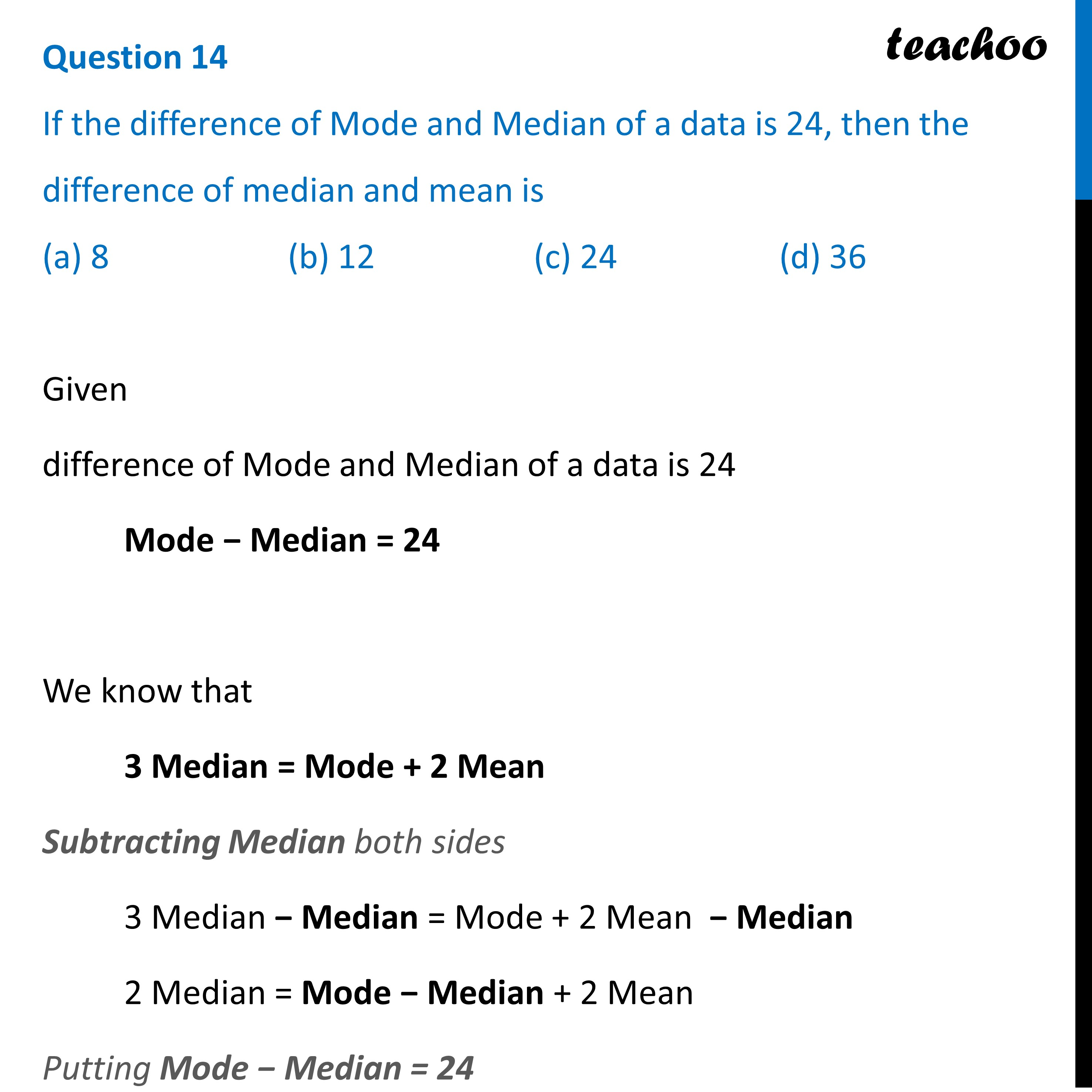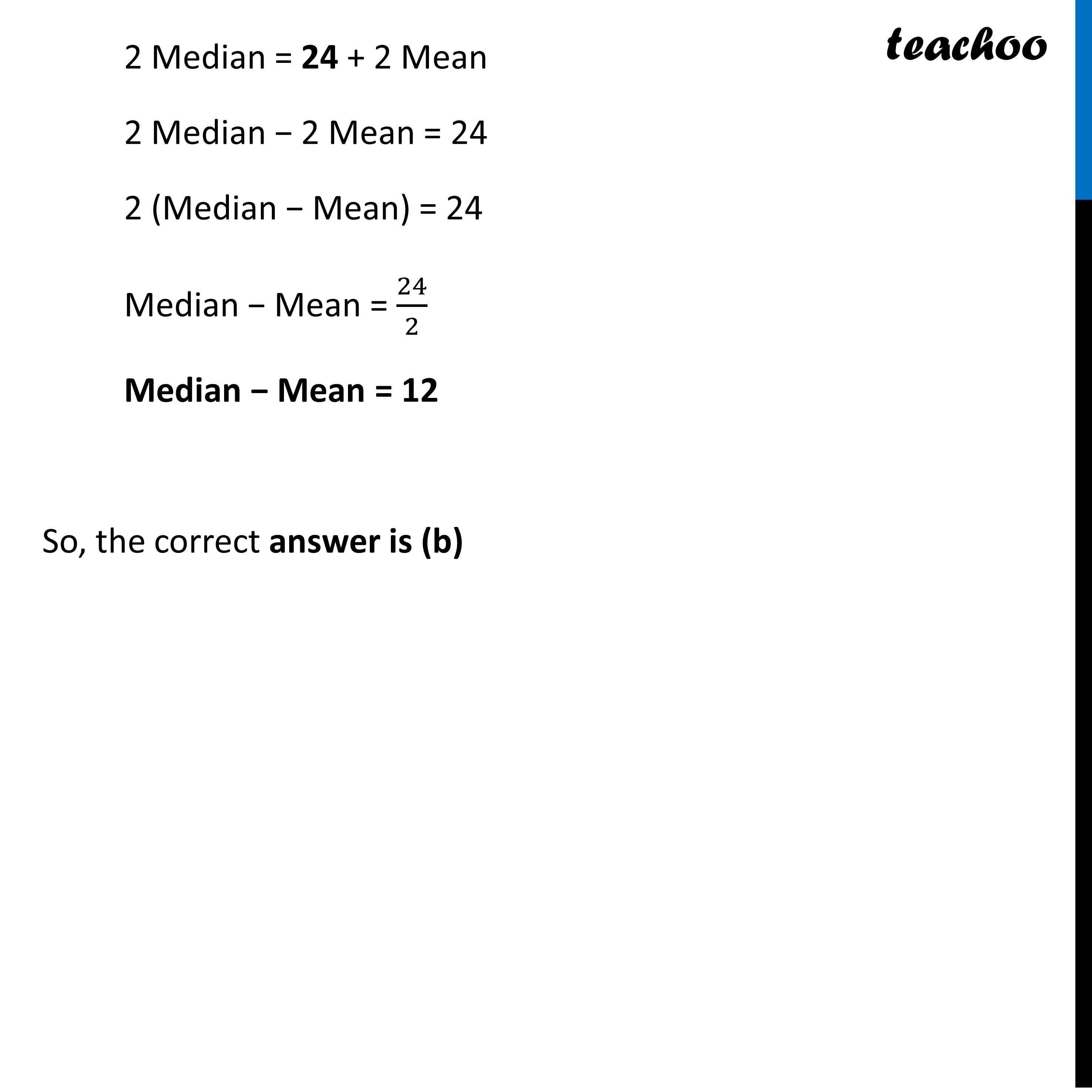CBSE Class 10 Sample Paper for 2023 Boards - Maths Standard

Class 10
Solutions of Sample Papers for Class 10 Boards

## (a) 8   (b) 12   (c) 24   (d) 36Get live Maths 1-on-1 Classs - Class 6 to 12

### Transcript

Question 14 If the difference of Mode and Median of a data is 24, then the difference of median and mean is (a) 8 (b) 12 (c) 24 (d) 36 Given difference of Mode and Median of a data is 24 Mode − Median = 24 We know that 3 Median = Mode + 2 Mean Subtracting Median both sides 3 Median − Median = Mode + 2 Mean − Median 2 Median = Mode − Median + 2 Mean Putting Mode − Median = 24 2 Median = 24 + 2 Mean 2 Median − 2 Mean = 24 2 (Median − Mean) = 24 Median − Mean = 24/2 Median − Mean = 12 So, the correct answer is (b)